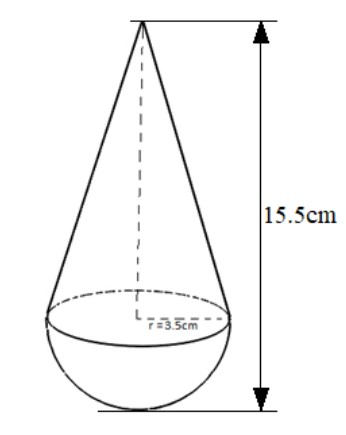QuestionAnswers

# A toy is in the form of a cone of radius $3.5cm$ mounted on a hemisphere of the same radius on its circular face. The total height of the tower is $15.5cm$. Find the total surface area of the toy.

Hint:Make a rough diagram with the information given in the question. Check for the areas that are exposed to you. Now calculate the area of the exposed parts of both cone and hemisphere using the formula for curved surface area.

Formula used:Curved surface area of the cone= $\pi r\sqrt {{r^2} + {h^2}}$ and Curved surface area of the hemisphere = $2\pi {r^2}$.
Let’s start everything with making a figure of what the question says.So, from the above figure it is clear that the total surface area of the toy which is exposed to us is the curved surface area of a cone and curved surface area of the hemisphere.
$\Rightarrow$ The total surface area of the toy$=$ Curved surface area of cone$+$ Curved surface area of hemisphere
Given the question that, radius$\left( r \right)$ of cone and hemisphere is $3.5cm$and the total height of toy, i.e. height of cone$+$ Radius of the hemisphere is $15.5cm$.
Height of the cone$\left( h \right) = 15.5 - 3.5 = 12cm$
We know that the formula for the curved surface area of the cone is $\pi$ times radius times slant height, i.e. $\pi r\sqrt {{r^2} + {h^2}}$ and for the hemisphere, the formula is twice the radius square times $\pi$, i.e. $2\pi {r^2}$
With these formulas, the above relation can be written as
The total surface area of the toy $= \pi r\sqrt {{r^2} + {h^2}} + 2\pi {r^2}$
Let us now substitute the known values in the equation
The total surface area of the toy $= \pi \times 3.5 \times \sqrt {{{3.5}^2} + {{12}^2}} + 2 \times \pi \times {3.5^2}$
Solving this equation with the value of $\pi = 3.141$
The total surface area of the toy$= 3.14 \times 3.5 \times \sqrt {12.25 + 144} + (2 \times 3.14 \times 12.25)$
On solving it further, you’ll get
The total surface area of the toy$= 10.99 \times \sqrt {156.25} + (2 \times 38.465) \Rightarrow 10.99 \times 12.5 + 76.93 \Rightarrow 137.375 + 76.93 \Rightarrow 214.3c{m^2}$.

Note:Try to understand what is asking first from the question.Always do the calculation step by step with proper use of braces. An alternative approach could be to find surface areas of both cone and hemisphere separately and then add them up to find the area of the toy.Students should remember formulas of surface areas of both cone and hemisphere for solving these types of questions.